Tag: quivers

Yesterday, Jan Stienstra gave a talk at theARTS entitled “Quivers, superpotentials and Dimer Models”. He started off by telling that the talk was based on a paper he put on the arXiv Hypergeometric Systems in two Variables, Quivers, Dimers and Dessins d’Enfants but that he was not going to say a thing about dessins but would rather focuss on the connection with superpotentials instead…pleasing some members of the public, while driving others to utter despair.

Anyway, it gave me the opportunity to figure out for myself what dessins might have to do with dimers, whathever these beasts are. Soon enough he put on a slide containing the definition of a dimer and from that moment on I was lost in my own thoughts… realizing that a dessin d’enfant had to be a dimer for the Dedekind tessellation of its associated Riemann surface!
and a few minutes later I could slap myself on the head for not having thought of this before :

There is a natural way to associate to a Farey symbol (aka a permutation representation of the modular group) a quiver and a superpotential (aka a necklace) defining (conjecturally) a Calabi-Yau algebra! Moreover, different embeddings of the cuboid tree diagrams in the hyperbolic plane may (again conjecturally) give rise to all sorts of arty-farty fanshi-wanshi dualities…

I’ll give here the details of the simplest example I worked out during the talk and will come back to general procedure later, when I’ve done a reference check. I don’t claim any originality here and probably all of this is contained in Stienstra’s paper or in some physics-paper, so if you know of a reference, please leave a comment. Okay, remember the Dedekind tessellation ?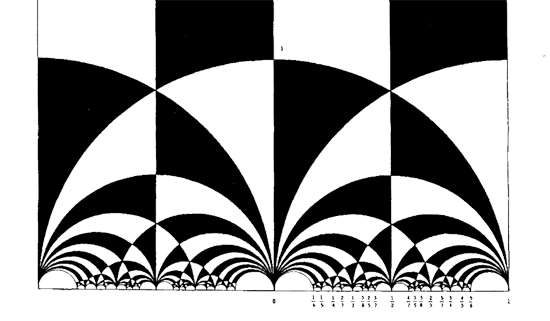So, all hyperbolic triangles we will encounter below are colored black or white. Now, take a Farey symbol and consider its associated special polygon in the hyperbolic plane. If we start with the Farey symbol

$$\xymatrix{\infty \ar@{-}_{(1)}[r] & 0 \ar@{-}_{\bullet}[r] & 1 \ar@{-}_{(1)}[r] & \infty}$$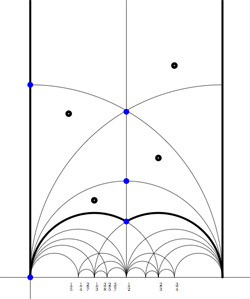we get the special polygonal region bounded by the thick edges, the vertical edges are identified as are the two bottom edges. Hence, this fundamental domain has 6 vertices (the 5 blue dots and the point at $i \infty$) and 8 hyperbolic triangles (4 colored black, indicated by a black dot, and 4 white ones).

Right, now let us associate a quiver to this triangulation (which embeds the quiver in the corresponding Riemann surface). The vertices of the triangulation are also the vertices of the quiver (so in our case we are going for a quiver with 6 vertices). Every hyperbolic edge in the triangulation gives one arrow in the quiver between the corresponding vertices. The orientation of the arrow is determined by the color of a triangle of which it is an edge : if the triangle is black, we run around its edges counter-clockwise and if the triangle is white we run over its edges clockwise (that is, the orientation of the arrow is independent of the choice of triangles to determine it). In our example, there is one arrows directed from the vertex at $i$ to the vertex at $0$, whether you use the black triangle on the left to determine the orientation or the white triangle on the right. If we do this for all edges in the triangulation we arrive at the quiver below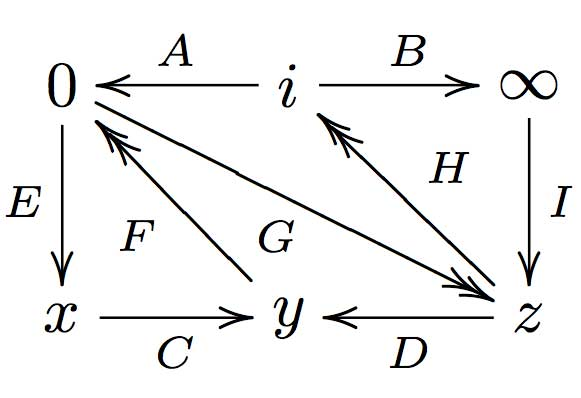where x,y and z are the three finite vertices on the $\frac{1}{2}$-axis from bottom to top and where I’ve used the physics-convention for double arrows, that is there are two F-arrows, two G-arrows and two H-arrows. Observe that the quiver is of Calabi-Yau type meaning that there are as much arrows coming into a vertex as there are arrows leaving the vertex.

Now that we have our quiver we determine the superpotential as follows. Fix an orientation on the Riemann surface (for example counter-clockwise) and sum over all black triangles the product of the edge-arrows counterclockwise MINUS sum over all white triangles
the product of the edge arrows counterclockwise. So, in our example we have the cubic superpotential

$IH’B+HAG+G’DF+FEC-BHI-H’G’A-GFD-CEF’$

From this we get the associated noncommutative algebra, which is the quotient of the path algebra of the above quiver modulo the following ‘commutativity relations’

$\begin{cases} GH &=G’H’ \\ IH’ &= IH \\ FE &= F’E \\ F’G’ &= FG \\ CF &= CF’ \\ EC &= GD \\ G’D &= EC \\ HA &= DF \\ DF’ &= H’A \\ AG &= BI \\ BI &= AG’ \end{cases}$

and morally this should be a Calabi-Yau algebra (( can someone who knows more about CYs verify this? )). This concludes the walk through of the procedure. Summarizing : to every Farey-symbol one associates a Calabi-Yau quiver and superpotential, possibly giving a Calabi-Yau algebra!

Take an affine $\mathbb{C}$-algebra A (not necessarily commutative). We will assign to it a strange object called the tangent-quiver $\vec{t}~A$, compute it in a few examples and later show how it connects with existing theory and how it can be used. This series of posts can be seen as the promised notes of my talks at the GAMAP-workshop but in reverse order… If some of the LaTeX-pictures are not in the desired spots, please size and resize your browser-window and they will find their intended positions.

A vertex $v$ of $\vec{t}~A$ corresponds to the isomorphism class of a finite dimensional simple A-representations $S_v$ and between any two such vertices, say $v$ and $w$, the number of directed arrows from $v$ to $w$ is given by the dimension of the Ext-space

$dim_{\mathbb{C}}~Ext^1_A(S_v,S_w)$

Recall that this Ext-space counts the equivalence classes of short exact sequences of A-representations

$$\xymatrix{0 \ar[r] & S_w \ar[r] & V \ar[r] & S_v \ar[r] & 0}$$

where two such sequences (say with middle terms V resp. W) are equivalent if there is an A-isomorphism $V \rightarrow^{\phi} W$ making the diagram below commutative

$$\xymatrix{0 \ar[r] & S_w \ar[r] \ar[d]^{id_{S_w}} & V \ar[r] \ar[d]^{\phi} & S_v \ar[r] \ar[d]^{id_{S_v}} & 0 \\\ 0 \ar[r] & S_w \ar[r] & W \ar[r] & S_v \ar[r] & 0}$$

The Ext-space measures how many non-split extensions there are between the two simples and is always a finite dimensional vectorspace. So the tangent quiver $\vec{t}~A$ has the property that in all vertices there are at most finitely many loops and between any two vertices there are a finite number of directed arrows, but in principle a vertex may be the origin of arrows connecting it to infinitely many other vertices.

Right, now let us at least motivate the terminology. Let $X$ be a (commutative) affine variety with coordinate ring $A = \mathbb{C}[X]$ then what is $\vec{t}~A$ in this case? To begin, as $\mathbb{C}[X]$ is commutative, all its finite dimensional simple representations are one-dimensional and there is one such for every point $x \in X$. Therefore, the vertices of $\vec{t}~A$ correspond to the points of the affine variety $X$. The simple A-representation $S_x$ corresponding to a point $x$ is just evaluating polynomials in $x$. Moreover, if $x \not= y$ then there are no non-split extensions between $S_x$ and $S_y$ (a commutative semi-local algebra splits as a direct sum of locals), therefore in $\vec{t}~A$ there can only be loops and no genuine arrows between different vertices. Finally, the number of loops in the vertex corresponding to the point $x$ can be computed using the fact that the self-extensions can be identified with the tangent space at $x$, that is

$dim_{\mathbb{C}}~Ext^1_{\mathbb{C}[X]}(S_x,S_x) = dim_{\mathbb{C}}~T_x~X$

That is, if $A=\mathbb{C}[X]$ is the coordinate ring of an affine variety $X$, then the quiver $\vec{t}~A$ is the set of points of $X$ having in each point $x$ as many loops as the dimension of the tangent space $T_x~X$. So, in this case, the quiver $\vec{t}~A$ contains all information about tangent spaces to the variety and that’s why we call it the tangent quiver.

Let’s go into the noncommutative wilderness. A first, quite trivial, example is the group algebra $A = \mathbb{C} G$ of a finite group $G$, then the simple A-representations are just the irreducible G-representations and as the group algebra is semi-simple every short exact sequence splits so all Ext-spaces are zero. That is, in this case the tangent quiver $\vec{t}~A$ in just a finite set of vertices (as many as there are irreducible G-representations) and no arrows nor loops.

Now you may ask whether there are examples of tangent quivers having arrows apart from loops. So, take another easy finite dimensional example : the path algebra $A = \mathbb{C} Q$ of a finite quiver $Q$ without oriented cycles. Recall that the path algebra is the vectorspace having as basis all vertices and all oriented paths in the quiver Q (and as there are no cycles, this basis is finite) and multiplication is induced by concatenation of paths. Here an easy example. Suppose the quiver Q looks like

$$\xymatrix{\vtx{} \ar[r] & \vtx{} \ar[r] & \vtx{}}$$

then the path algebra is 6 dimensional as there are 3 vertices, 2 paths of length one (the arrows) and one path of length two (going from the leftmost to the rightmost vertex). The concatenation rule shows that the three vertices will give three idempotents in A and one easily verifies that the path algebra can be identified with upper-triangular $3 \times 3$ matrices

$\mathbb{C} Q \simeq \begin{bmatrix} \mathbb{C} & \mathbb{C} & \mathbb{C} \\\ 0 & \mathbb{C} & \mathbb{C} \\\ 0 & 0 & \mathbb{C} \end{bmatrix}$

where the diagonal components correspond to the vertices, the first offdiagonal components to the two arrows and the corner component corresponds to the unique path of length two. Right, for a general finite quiver without oriented cycles is the quite easy to see that all finite dimensional simples are one-dimensional and correspond to the vertex-idempotents, that is every simple is of the form $S_v = e_v \mathbb{C} Q e_v$ where $e_v$ is the vertex idempotent. No doubt, you can guess what the tangent quiver $\vec{t}~A = \vec{t}~\mathbb{C} Q$ will be, can’t you?

The categorical cafe has a guest post by Tom Leinster Linear Algebra Done Right on the book with the same title by Sheldon Axler. I haven’t read the book but glanced through his online paper Down with determinants!. Here is ‘his’ proof of the fact that any n by n matrix A has at least one eigenvector. Take a vector $v \in \mathbb{C}^n$, then as the collection of vectors ${ v,A.v,A^2.v,\ldots,A^n.v }$ must be linearly dependent, there are complex numbers $a_i \in \mathbb{C}$ such that $~(a_0 + a_1 A + a_2 A^2 + \ldots + a_n A^n).v = \vec{0} \in \mathbb{C}^n$ But then as $\mathbb{C}$ is algebraically closed the polynomial on the left factors into linear factors $a_0 + a_1 x + a_2 x^2 + \ldots + a_n x^n = c (x-r_1)(x-r_2) \ldots (x-r_n)$ and therefore as $c(A-r_1I_n)(A-r_2I_n) \ldots (A-r_nI_n).v = \vec{0}$ from which it follows that at least one of the linear transformations $A-r_j I_n$ has a non-trivial kernel, whence A has an eigenvector with eigenvalue $r_j$. Okay, fine, nice even, but does this simple minded observation warrant the extreme conclusion of his paper (on page 18) ?

As mathematicians, we often read a nice new proof of a known theorem, enjoy the different approach, but continue to derive our internal understanding from the method we originally learned. This paper aims to change drastically the way mathematicians think about and teach crucial aspects of linear algebra.

The simple proof of the existence of eigenvalues given in Theorem 2.1 should be the one imprinted in our minds, written on our blackboards, and published in our textbooks. Generalized eigenvectors should become a central tool for the understanding of linear operators. As we have seen, their use leads to natural definitions of multiplicity and the characteristic polynomial. Every mathematician and every linear algebra student should at least remember that the generalized eigenvectors of an operator always span the domain (Proposition 3.4)‚Äîthis crucial result leads to easy proofs of upper-triangular form (Theorem 6.2) and the Spectral Theorem (Theorems 7.5 and 8.3).

Determinants appear in many proofs not discussed here. If you scrutinize such proofs, you‚Äôll often discover better alternatives without determinants. Down with Determinants!

I welcome all new proofs of known results as they allow instructors to choose the one best suited to their students (and preferable giving more than one proof showing that there is no such thing as ‘the best way’ to prove a mathematical result). What worries me is Axler’s attitude shared by extremists and dogmatics world-wide : they are so blinded by their own right that they impoverish their own lifes (and if they had their way, also that of others) by not willing to consider other alternatives. A few other comments :

1. I would be far more impressed if he had given a short argument for the one line he skates over in his proof, that of $\mathbb{C}$ being algebraically closed. Does anyone give a proof of this fact anymore or is this one of the few facts we expect first year students to accept on faith?

1. I dont understand this aversity to the determinant (probably because of its nonlinear character) but at the same time not having any problems with successive powers of matrices. Surely he knows that the determinant is a fixed $~\mathbb{Q}~$-polynomial in the traces (which are linear!) of powers of the matrix.

2. The essense of linear algebra is that by choosing a basis cleverly one can express a linear operator in a extremely nice matrix form (a canonical form) so that all computations become much more easy. This crucial idea of considering different bases and their basechange seems to be missing from Axler’s approach. Moreover, I would have thought that everyone would know these days that ‘linear algebra done right’ is a well developed topic called ‘representation theory of quivers’ but I realize this might be viewed as a dogmatic statement. Fortunately someone else is giving the basic linear algebra courses here in Antwerp so students are spared my private obsessions (at least the first few years…). In [his post](http://golem.ph.utexas.edu/category/2007/05/ linear_algebra_done_right.html) Leistner askes “What are determinants good for?” I cannot resist mentioning a trivial observation I made last week when thinking once again about THE rationality problem and which may be well known to others. Recall from the previous post that rationality of the quotient variety of matrix-couples $~(A,B) \in M_n(\mathbb{C}) \oplus M_n(\mathbb{C}) / GL_n$ under _simultaneous conjugation_ is a very hard problem. On the other hand, the ‘near miss’ problem of the quotient variety of matrix-couples ${ (A,B)~|~det(A)=0~} / GL_n$ is completely trivial. It is rational for all n. Here is a one-line proof. Consider the quiver $\xymatrix{\vtx{} \ar@/^2ex/[rr] & & \vtx{} \ar@(ur,dr) \ar@/^2ex/[ll]}$ then the dimension vector (n-1,n) is a Schur root and the first fundamental theorem of $GL_n$ (see for example Hanspeter Krafts excellent book on invariant theory) asserts that the corresponding quotient variety is the one above. The result then follows from Aidan Schofield’s paper Birational classification of moduli spaces of representations of quivers. Btw. in this special case one does not have to use the full force of Aidan’s result. Zinovy Reichstein, who keeps me updated on events in Atlanta, emailed the following elegant short proof Here is an outline of a geometric proof. Let $X = {(A, B) : det(A) = 0} \subset M_n^2$ and $Y = \mathbb{P}^{n-1} \times M_n$. Applying the no-name lemma to the $PGL_n$-equivariant dominant rational map $~X \rightarrow Y$ given by $~(A, B) \rightarrow (Ker(A), B)$ (which makes X into a vector bundle over a dense open $PGL_n$-invariant subset of Y), we see that $X//PGL_n$ is rational over $Y//PGL_n$ On the other hand, $Y//PGLn = M_n//PGL_n$ is an affine space. Thus $X//PGL_n$ is rational. The moment I read this I knew how to do this quiver-wise and that it is just another Brauer-Severi type argument so completely inadequate to help settling the genuine matrix-problem. Update on the paper by Esther Beneish : Esther did submit the paper in february.

After a lengthy spring-break, let us continue with our course on noncommutative geometry and $SL_2(\mathbb{Z})$-representations. Last time, we have explained Grothendiecks mantra that all algebraic curves defined over number fields are contained in the profinite compactification
$\widehat{SL_2(\mathbb{Z})} = \underset{\leftarrow}{lim}~SL_2(\mathbb{Z})/N$ of the modular group $SL_2(\mathbb{Z})$ and in the knowledge of a certain subgroup G of its group of outer automorphisms
. In particular we have seen that many curves defined over the algebraic numbers $\overline{\mathbb{Q}}$ correspond to permutation representations of $SL_2(\mathbb{Z})$. The profinite compactification $\widehat{SL_2}=\widehat{SL_2(\mathbb{Z})}$ is a continuous group, so it makes sense to consider its continuous n-dimensional representations $\mathbf{rep}_n^c~\widehat{SL_2}$ Such representations are known to have a finite image in $GL_n(\mathbb{C})$ and therefore we get an embedding $\mathbf{rep}_n^c~\widehat{SL_2} \hookrightarrow \mathbf{rep}_n^{ss}~SL_n(\mathbb{C})$ into all n-dimensional (semi-simple) representations of $SL_2(\mathbb{Z})$. We consider such semi-simple points as classical objects as they are determined by – curves defined over $\overline{Q}$ – representations of (sporadic) finite groups – modlart data of fusion rings in RCTF – etc… To get a feel for the distinction between these continuous representations of the cofinite completion and all representations, consider the case of $\hat{\mathbb{Z}} = \underset{\leftarrow}{lim}~\mathbb{Z}/n \mathbb{Z}$. Its one-dimensional continuous representations are determined by roots of unity, whereas all one-dimensional (necessarily simple) representations of $\mathbb{Z}=C_{\infty}$ are determined by all elements of $\mathbb{C}$. Hence, the image of $\mathbf{rep}_1^c~\hat{\mathbb{Z}} \hookrightarrow \mathbf{rep}_1~C_{\infty}$ is contained in the unit circle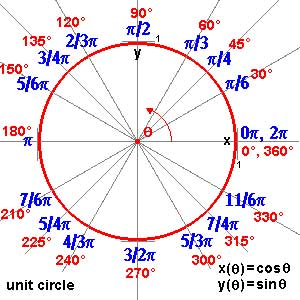and though these points are very special there are enough of them (technically, they form a Zariski dense subset of all representations). Our aim will be twofold : (1) when viewing a classical object as a representation of $SL_2(\mathbb{Z})$ we can define its modular content (which will be the noncommutative tangent space in this classical point to the noncommutative manifold of $SL_2(\mathbb{Z})$). In this way we will associate noncommutative gadgets to our classical object (such as orders in central simple algebras, infinite dimensional Lie algebras, noncommutative potentials etc. etc.) which give us new tools to study these objects. (2) conversely, as we control the tangentspaces in these special points, they will allow us to determine other $SL_2(\mathbb{Z})$-representations and as we vary over all classical objects, we hope to get ALL finite dimensional modular representations. I agree this may all sound rather vague, so let me give one example we will work out in full detail later on. Remember that one can reconstruct the sporadic simple Mathieu group $M_{24}$ from the dessin d’enfant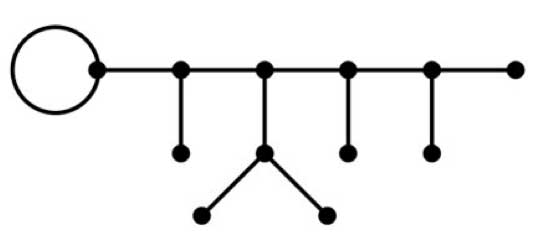This
dessin determines a 24-dimensional permutation representation (of
$M_{24}$ as well of $SL_2(\mathbb{Z})$) which
decomposes as the direct sum of the trivial representation and a simple
23-dimensional representation. We will see that the noncommutative
tangent space in a semi-simple representation of
$SL_2(\mathbb{Z})$ is determined by a quiver (that is, an
oriented graph) on as many vertices as there are non-isomorphic simple
components. In this special case we get the quiver on two points
$\xymatrix{\vtx{} \ar@/^2ex/[rr] & & \vtx{} \ar@/^2ex/[ll] \ar@{=>}@(ur,dr)^{96} }$ with just one arrow in each direction
between the vertices and 96 loops in the second vertex. To the
experienced tangent space-reader this picture (and in particular that
there is a unique cycle between the two vertices) tells the remarkable
fact that there is **a distinguished one-parameter family of
24-dimensional simple modular representations degenerating to the
permutation representation of the largest Mathieu-group**. Phrased
differently, there is a specific noncommutative modular Riemann surface
associated to $M_{24}$, which is a new object (at least as far
as I’m aware) associated to this most remarkable of sporadic groups.
Conversely, from the matrix-representation of the 24-dimensional
permutation representation of $M_{24}$ we obtain representants
of all of this one-parameter family of simple
$SL_2(\mathbb{Z})$-representations to which we can then perform
noncommutative flow-tricks to get a Zariski dense set of all
24-dimensional simples lying in the same component. (Btw. there are
also such noncommutative Riemann surfaces associated to the other
is what we will be doing in the upcoming posts (10) : explain what a
noncommutative tangent space is and what it has to do with quivers (11)
what is the noncommutative manifold of $SL_2(\mathbb{Z})$? and what is its connection with the Kontsevich-Soibelman coalgebra? (12)
is there a noncommutative compactification of $SL_2(\mathbb{Z})$? (and other arithmetical groups) (13) : how does one calculate the noncommutative curves associated to the Mathieu groups? (14) : whatever comes next… (if anything).

Next
week our master programme on noncommutative geometry
will start. Here is the list of all international mini-courses (8 hours
each) and firm or tentative dates. For the latest update, it is always
best to check with the Arts seminar
website
.

• Hans-Juergen Schneider (Munich) “Hopf Galois extensions and
quotient theory of Hopf algebras”. February 20-23 each day from
10h30-12h30.

• Markus Reineke
(Wuppertal) “Representations of quivers”. February 27-28, March 1-2
each day from 10h30-12h30.

• Arthur Ruuge
(Moscow) “Semiclassical approximation of quantum
mechanics”. March 6-9 each day from 10h30-12h30.
• Rupert Yu
(Poitiers) in March or April.
• Isar Stubbe (Antwerp) in April.
• Fred Van Oystayen (Antwerp) in April.
• Raf
Bocklandt (Antwerp) in April or May.
• Goro Kato (Los Angeles)
in May.
• Florin Panaite (Bucharest) in May.
• Pjotr
Hajac (Warsaw) in June.

Apart from these mini-courses
there will be four regular courses (approx. 30hrs each) during the whole
semester.

• Raf Bocklandt “Knot theory”.
• Lieven Le Bruyn “Noncommutative geometry”.
• Geert Van
de Weyer “Quantum groups”.
• Fred Van Oysyaeyen
“Noncommutative algebra”.

<

p>Dates and places of all
lectures will be made available through the Arts seminar
site
.